# Radiation effects on Williamson nanofluid flow over a heated surface with magnetohydrodynamics

Radiation effects on Williamson nanofluid flow over a heated surface with magnetohydrodynamics

Fazle Mabood Sheikh M. Ibrahim Giulio LorenziniEnrico Lorenzini

Department of Mathematics, University of Peshawar, Peshawaar 25120, Pakistan

Department of Mathematics, GITAM University, Visakhapatnam, Andhra Pradesh, India – 530045

Department of Engineering and Architecture, University of Parma, Parco Area delle Scienze 181/A, 43124 Parma, Italy.

Alma Mater Studiorum-University of Bologna, Department of Industrial Engineering, viale Risorgimento no.2, Bologna 40136, Italy

Corresponding Author Email:
giulio.lorenzini@unipr.it
Page:
196-204
|
DOI:
https://doi.org/10.18280/ijht.350126
|
Accepted:
|
Published:
31 March 2017
| Citation

OPEN ACCESS

Abstract:

An analysis is performed for magnetohydrodynamics (MHD) Williamson nanofluid flow over a continuously moving heated surface with thermal radiation and heat source. The governing partial differential equations are transformed into self-similar ordinary differential equations using similarity transformations. The obtained self-similar equations are solved by finite difference method using quasilinearization technique. Mathematical analysis of various physical parameters is presented in graphs. The impact of physical parameters on skin-friction coefficient, reduced Nusselt and Sherwood numbers are shown in tabular and graphical form. Furthermore, comparison of the present analysis is made with the previously existing literature and an appreciable agreement in the values is observed for the limiting case.

Keywords:

Nanofluid, MHD, Radiation, Heat Source, Non-Linearly Moving Surface

1. Introduction

Magneto-fluid-dynamics or hydro-magnetics is a field of research which analyzes the dynamics of electricity conducting fluids such as plasmas, liquid metals and salt water. The term magnetohydrodynamics (MHD) is a combination of magneto (magnetic field), hydro (liquid) and dynamics (movement of particles). The magnetic field induced current flows in a dynamic fluid and creates forces on the fluid. Some important applications of radiative heat transfer include MHD accelerators, high temperature plasmas, power generation systems and cooling of nuclear reactors. Many processes in engineering areas occur at high temperatures and knowledge of radiation heat transfer becomes very important for the design of pertinent equipment. The heat transfer analysis of boundary layer flow with radiation is also important in electrical power generation, astrophysical flows, solar power technology, space vehicle re-entry and other industrial areas. Some significant applications of MHD flow and/or heat transfer over a stretching sheet can be found in [1-6].

A nanofluid is the combination of simple fluid and nano-sized particles uniformly suspended in the fluid. The nanoparticles used in nanofluids are typically made of metals (Al, Cu), oxides (Al2O3), carbides (SiC), nitrides (AlN, SiN) or nonmetals (graphite, carbon nanotubes) and the base fluid is usually a conductive fluid, such as water (as in this study) or ethylene glycol. Nanoparticles range in diameter between 1-100 nm. Experimental studies have shown that nanofluids commonly need only contain up to a 5% volume fraction of nanoparticles to ensure effective heat transfer enhancements . Nanofluids offer many diverse advantages in application such as microelectronics, fuel cell, nuclear reactors, biomedicine and transportation. Important work on the boundary layer flow of a nanofluid over a stretching sheet has been reported by Khan and Pop , Rana and Bhargava  conducted similar research for a nonlinear stretching sheet, Mabood et al.  reported MHD boundary layer flow and heat transfer of nanofluids over a nonlinear stretching sheet. Numerous researchers [11-16] reported related studies for linear/nonlinear stretching sheet.

Boundary layer flow of non-Newtonian fluids [17-23] has gained much attention of the recent researchers. It is because of its wide applications in the industrial and engineering processes. In non-Newtonian fluids, the most commonly encountered fluids are pseudoplastic fluids. The study of the boundary layer flow of pesudoplastic fluids is of great interest due to its wide range of application in industry such as extrusion of polymer sheets, emulsion coated sheets like photographic films, solutions and melts of high molecular weight polymers, etc. To explain the behaviour of pseudoplastic fluids many models have been proposed like the power law model, Carreaus model, Cross model and Ellis model, but little attention has been paid to the Williamson fluid model. Williamson  discussed the flow of pseudoplastic materials and proposed a model equation to describe the flow of pseudoplastic fluids and experimentally verified the results. Irene and Giambattista  obtained perturbation solutions for pulsatile flow of a non-Newtonian Williamson fluid in a rock fracture. Influence of inclined magnetic field on peristaltic flow of Williamson fluid model in an inclined symmetric or asymmetric channel reported by Nadeem et al. . Vajravelu et al.  investigated the peristaltic transport of a Williamson fluid in asymmetric channels with permeable walls. Later, Nadeem et al.  discussed the peristaltic flow of a Williamson fluid in an asymmetric channel.

It is evident from the previous literature works that no attempt has been done by the researchers to analyze the flow behavior of Williamson nanofluid in the presence of radiation and heat source. The relevant boundary layer equations are modeled for Williamson nanofluid.  In order to examine the validity of results they are compared with the published data for some special cases. Effects of important physical parameters have been discussed through graphs and tables.

2. Mathematical Formulation

We consider a steady two dimensional flow of an incompressible MHD Williamson nanofluid over a horizontal heated surface. Where x and y- axis are taken along horizontal and vertical direction respectively, so that nanofluids confined to y>0. The plate is stretched along x-axis with the velocity $a{{x}^{n}}$, where a>0 is stretching parameter and n is the stretching index. It is assumed that the surface is heated due to convection of hot fluid below the surface. A space varying magnetic field $B(0,B(x),0)$ is applied along the transverse direction of the flow. The fluid is assumed to be slightly conducting, so that the magnetic Reynolds number is much less that unity and hence the induced magnetic field is negligible in comparison to the applied magnetic field. The general transport equations for nanofluid are given by Buongiorno 

div V=0,      (1)

$\rho \frac{dV}{dt}=divS+\rho b,$      (2)

$\rho c\left( \frac{\partial T}{\partial t}+V.\nabla T \right)=\nabla .k\nabla T+{{\rho }_{p}}{{c}_{p}}\left( {{D}_{B}}\nabla \varphi .\nabla T+{{D}_{T}}\frac{\nabla T.\nabla T.}{{{T}_{\infty }}} \right),$      (3)

$\frac{\partial C}{\partial t}+V.\nabla C=\nabla .\left( {{D}_{B}}\nabla C+{{D}_{T}}\frac{\nabla T}{{{T}_{\infty }}} \right),$         (4)

where in steady state the velocity vector is given by V(u(x,y), v(x,y),0), $\rho$ is nanofluid density, ${{\rho }_{p}}$ is nanoparticle density, S is Cauchy stress tensor, b is body force vector, c is the heat capacity of nanofluid, cp is heat capacity of nanoparticles, T  is temperature, k is nanofluid thermal conductivity, DB is Brownian diffusion coefficient, C is nanoparticle volumetric fraction, DT is thermophoretic diffusion coefficient and ${{T}_{\infty }}$ is ambient fluid temperature. For Williamson fluid model Cauchy stress tensor S is defined in (Dapra ) as

$S=-pI+\tau ,$      (5)

$\tau =\left( {{\mu }_{\infty }}+\frac{\left( {{\mu }_{0}}-{{\mu }_{\infty }} \right)}{1-\Gamma \dot{\gamma }} \right){{A}_{1}},$       (6)

In above equations, $\tau$ is extra stress tensor, ${{\mu }_{0}}$ is limiting viscosity at zero shear rate and ${{\mu }_{\infty }}$ is limiting viscosity at infinite shear rate, $\Gamma >0$ is a time constant, A1 is the first Rivlin-Erickson tensor and $\dot{\gamma }$ is defined as

$\dot{\gamma }=\sqrt{\frac{1}{2}\pi },$          (7)

where $\pi =trace(A_{1}^{2})$

Here, we considered the case for which ${{\mu }_{\infty }}=0$ and $\Gamma \dot{\gamma }<1.$ Thus equation (6) can be written as

$\tau =\left( \frac{{{\mu }_{0}}}{1-\Gamma \dot{\gamma }} \right){{A}_{1}},$          (8)

or by using binomial expansion we get

$\tau ={{\mu }_{0}}\left( 1+\Gamma \dot{\gamma } \right){{A}_{1}},$          (9)

The interaction of magnetic field and velocity will give rise to the Lorentz force JxB defined in equation (10), in which J is the current density and $\sigma$ is the electrical conductivity. In the absence of electric field E = 0.

$J=\sigma \left( E+V\times B \right)$      (10)

Since we have considered the steady state velocity so all the derivatives w.r.t are zero. Making use of equations (5), (9) and (10) in equations (1)–(4) two dimensional boundary layer equations governing the flow are given by

$\frac{\partial u}{\partial x}+\frac{\partial v}{\partial y}=0,$         (11)

$u\frac{\partial u}{\partial x}+v\frac{\partial u}{\partial y}=\nu \frac{{{\partial }^{2}}u}{\partial {{y}^{2}}}+\sqrt{2}\nu \Gamma \frac{\partial u}{\partial y}\frac{{{\partial }^{2}}u}{\partial {{y}^{2}}}-\frac{\sigma {{B}^{2}}(x)u}{\rho },$       (12)

$u\frac{\partial T}{\partial x}+v\frac{\partial T}{\partial y}=\alpha \frac{{{\partial }^{2}}T}{\partial {{y}^{2}}}\,+\frac{{{\rho }_{p}}{{c}_{p}}}{\rho c}\left( {{D}_{B}}\frac{\partial C}{\partial y}\frac{\partial T}{\partial y}+\frac{{{D}_{T}}}{{{T}_{\infty }}}{{\left( \frac{\partial T}{\partial y} \right)}^{2}} \right)-\frac{1}{{{\rho }_{p}}{{c}_{p}}}\frac{\partial {{q}_{r}}}{\partial y}+\frac{{{Q}^{*}}}{{{\rho }_{p}}{{c}_{p}}}\left( T-{{T}_{\infty }} \right),$.    (13)

$u\frac{\partial C}{\partial x}+v\frac{\partial C}{\partial y}={{D}_{B}}\frac{{{\partial }^{2}}C}{\partial {{y}^{2}}}+\frac{{{D}_{T}}}{{{T}_{\infty }}}\frac{{{\partial }^{2}}T}{\partial {{y}^{2}}},$       (14)

where u(x,y) and v(x,y) are horizontal and vertical components of velocity, $\nu$ is kinematic viscosity and $\alpha$ is nanofluid thermal diffusivity. The magnetic field is chosen as $B(x)={{B}_{0}}\sqrt{{{x}^{n-1}}}$. The corresponding boundary conditions to the flow problem are

$u={{U}_{w}}=a{{x}^{n}};$  v = 0; $-k\frac{\partial T}{\partial y}=h\left( {{T}_{f}}-T \right),$ $C={{C}_{w}}$

at  y =0, $u\to 0;$  $T={{T}_{\infty }}$; $C={{C}_{\infty }}$  as $y\to \infty$          (15)

Using Rosseland approximation for radiation, the radiative heat flux is simplified as,

${{q}_{r}}=-\frac{4{{\sigma }^{*}}}{3{{k}^{*}}}\frac{\partial {{T}^{4}}}{\partial y}$        (16)

where σ* is Stefan–Boltzmann constant and k* the mean absorption coefficient. The temperature differences within the flow are assumed to be small enough so that T4 may be expressed as a linear function of temperature T using a truncated Taylor series about the free stream temperature ${{T}_{\infty }}$, and neglecting the higher order terms we get,

${{T}^{4}}\simeq 4TT_{\infty }^{3}-3T_{\infty }^{3}$          (17)

Substituting (16) and (17) in (13), we have

\begin{align} & u\frac{\partial T}{\partial x}+v\frac{\partial T}{\partial y}=\alpha \frac{{{\partial }^{2}}T}{\partial {{y}^{2}}}+\frac{{{\rho }_{p}}{{c}_{p}}}{\rho c}\left( {{D}_{B}}\frac{\partial C}{\partial y}\frac{\partial T}{\partial y}+\frac{{{D}_{T}}}{{{T}_{\infty }}}{{\left( \frac{\partial T}{\partial y} \right)}^{2}} \right) \\ & \,\,\,\,\,\,\,\,\,\,\,\,\,\,\,\,-\frac{16{{\sigma }^{*}}T_{\infty }^{3}}{3{{k}^{*}}{{\rho }_{p}}{{c}_{p}}}\frac{{{\partial }^{2}}T}{\partial {{y}^{2}}}+\frac{{{Q}^{*}}}{{{\rho }_{p}}{{c}_{p}}}\left( T-{{T}_{\infty }} \right), \\\end{align}      (18)

In boundary conditions k is thermal conductivity, Tf is the temperature of the hot fluid and h is the convective heat transfer coefficient. Introducing the following transformations in above equations

$u=a{{x}^{n}}{f}'(\eta )$, $v=-\sqrt{\frac{a(n+1)\nu {{x}^{n-1}}}{2}}\left( f(\eta )+\frac{n-1}{n+1}\eta {f}'(\eta ) \right)$,$\eta =\sqrt{\frac{a(n+1){{x}^{n-1}}}{2\nu }},\,\,\,\theta =\frac{T-{{T}_{\infty }}}{{{T}_{w}}-{{T}_{\infty }}},\,\,\,\phi =\frac{C-{{C}_{\infty }}}{{{C}_{w}}-{{C}_{\infty }}}$        (19)

With the help of above transformations, equation (11) is identically satisfied and equations (12) (14) and (18) along with boundary conditions (15) take the following form

\begin{align} & {f}'''(\eta )-\frac{2n}{n+1}{{{{f}'}}^{2}}(\eta )+f(\eta ){f}''(\eta )\,+\lambda {f}''(\eta ){f}'''(\eta ) \\ & \,\,\,\,\,\,\,\,\,\,\,\,\,\,\,\,\,\,\,\,\,\,\,\,\,\,\,\,\,\,\,\,\,\,\,\,\,\,\,\,\,\,\,\,\,\,\,\,\,\,\,\,\,\,\,\,\,\,\,\,\,\,\,\,\,\,\,\,\,\,\,\,\,\,\,\,\,\,\,-M{f}'(\eta )=0, \\\end{align}         (20)

\begin{align} & \left( 1+R \right){\theta }''(\eta )+\Pr f(\eta ){\theta }'(\eta )+\Pr Q\theta (\eta ) \\ & \,\,\,\,\,\,\,\,\,\,\,\,\,\,\,\,\,\,\,\,\,\,\,\,\,\,\,\,\,\,+\frac{Nc}{Le}{\phi }'(\eta ){\theta }'(\eta )+\frac{Nc}{LeNbt}{{{{\theta }'}}^{2}}(\eta )=0, \\\end{align}          (21)

${\phi }''(\eta )+Scf(\eta ){\phi }'(\eta )+\frac{1}{Nbt}{\theta }''(\eta )=0$        (22)

Mathematically, Schmidt number Sc can be written as

$Sc=\frac{\upsilon }{{{D}_{B}}}=\frac{\alpha }{{{D}_{B}}}\frac{\upsilon }{\alpha }=Le.\Pr$          (23)

With the help of equation (23), equation (22) can be written as

${\phi }''(\eta )+Le\Pr f(\eta ){\phi }'(\eta )+\frac{1}{Nbt}{\theta }''(\eta )=0$         (24)

The corresponding boundary conditions are

$f=0,\,\,{f}'=1,\,\,{\theta }'=-Bi\left( 1-\theta \right),\,\,\phi =1$at $\eta =0$         (25)

${f}'=0,$   $\theta =0,$     $\phi =0$     as     $\eta \to \infty$.

Since we are interested in the study of heat transfer enhancement, so it is better to introduce the effects of thermal diffusivity in nanoparticle equation with the help of Le and Pr. Also it will enhance the coupling effects of heat and nanoparticle equation. Finally, equations (17), (18) and (21) form the non-dimensional system of equation with corresponding boundary equations given in (22). For $\lambda =0$, problem reduces to the one for Newtonian nanofluid and for DB = DT = 0 in equation (13), heat equation reduces to the classical boundary layer heat equation in the absence of viscous dissipation. Physical quantities of interest for the present study are skin-friction coefficient, reduced Nusselt number Nu and local Sherwood number Sh.

$Nu=\frac{-x}{{{T}_{w}}-{{T}_{\infty }}}{{\left( \frac{\partial T}{\partial y} \right)}_{y=0}}$, $Sh=\frac{-x}{{{C}_{w}}-{{C}_{\infty }}}{{\left( \frac{\partial C}{\partial y} \right)}_{y=0}}$         (26)

or by introducing the transformations (16), we get

$\frac{Nu}{\sqrt{{{\operatorname{Re}}_{x}}}}=-\sqrt{\frac{n+1}{2}}{\theta }'(0),$ $\frac{Sh}{\sqrt{{{\operatorname{Re}}_{x}}}}=-\sqrt{\frac{n+1}{2}}{\phi }'(0),$          (27)

where

${{\operatorname{Re}}_{_{x}}}=\frac{{{U}_{w}}x}{\upsilon }$ is local Reynolds number,

$\lambda =\Gamma \sqrt{\frac{{{a}^{3}}(n+1){{x}^{3n-1}}}{\nu }}$ (Non Newtonian Williamson parameter),

$\Pr =\frac{\upsilon }{\alpha }$(Prandtl number = momentum diffusivity/nanofluid thermal diffusivity),

$R=\frac{4{{\sigma }^{*}}T_{\infty }^{3}}{k{{k}^{*}}}$(Radiation parameter)

${{Q}^{*}}=\frac{Q}{{{\rho }_{p}}{{c}_{p}}a}$ (Heat source parameter)

$Le=\frac{\alpha }{{{D}_{B}}}$(Lewis number = nanofluid thermal diffusivity/Brownian diffusivity),

$Sc=\frac{\upsilon }{{{D}_{B}}}$ (Schmidt number = momentum diffusivity / Brownian diffusivity),

$M=\frac{2\sigma B_{0}^{2}}{\rho a(n+1)}$ (Magnetic field parameter),

$Nc=\frac{{{\rho }_{p}}{{c}_{p}}}{\rho c}\left( {{C}_{w}}-{{C}_{\infty }} \right)$ (Heat capacities ratio = nanoparticles heat capacity/nanofluid heat capacity),

$Nbt=\frac{{{D}_{B}}{{T}_{\infty }}\left( {{C}_{w}}-{{C}_{\infty }} \right)}{{{D}_{T}}\left( {{T}_{w}}-{{T}_{\infty }} \right)}$ (Diffusivity ratio = Brownian diffusivity /thermophoretic diffusivity),

$\frac{Bi}{\sqrt{{{\operatorname{Re}}_{x}}}}=\frac{hx}{k}\sqrt{\frac{2}{n+1}}$ (Biot number).

3. Numerical Method

Equations (20), (21) and (24) subject to the boundary conditions (25) were solved numerically using an implicit finite difference method with quasi-linearization technique. The details of the method can be found in Inoyue and Tate , and Bellman and Kalaba . The effects of the emerging parameters on the dimensionless velocity, temperature, concentration, and, heat and mass transfer rates are investigated. The step size and convergence criteria were taken as $\Delta \eta =0.001$ and 10-6 respectively. The asymptotic boundary conditions in equation (25) were approximated by using a value of 10 for the similarity variable ${{\eta }_{\max }}$as follows

${{\eta }_{\max }}=10$,${f}'\left( 10 \right)=\theta \left( 10 \right)=\phi \left( 10 \right)=0$.         (28)

The choice of ${{\eta }_{\max }}=10$ ensures that all numerical solutions approached the asymptotic values correctly.

4. Results and Discussion

In order to investigate the physical representation of the problem, the numerical values of velocity $\left( {f}'(\eta ) \right)$, temperature $\left( \theta (\eta ) \right)$ and concentration $\left( \phi (\eta ) \right)$ have been computed for resultant principal parameters as the magnetic field parameter M, non-Newtonian Williamson parameter $\lambda$, stretching index parameter n, Biot parameter Bi, heat source parameter Q, radiation parameter R, Lewis number Le, heat capacities ratio Nc and diffusivity ratio Nbt respectively. In order to test the accuracy of the present method, our results are compared with those of Rana and Bhargava , Cortell , Zaimi et al. , Khan and Pop , Wang  and Gorla and Sidawi  (for reduced cases) and noticed that there is an excellent agreement, as shown in tables 1 and 2. Throughout the calculations, the parametric values are fixed to be M = 1.0, n = 0.5, $\lambda =Nc=R=Q=0.2$, Nbt = Le = 2.0, Pr = 6.2, Bi = 1.0, unless otherwise indicated.

Figure 1 is drawn to examine the impact of magnetic field parameter M on the nanofluid velocity. It is observed that, the velocity profile decrease with increase in magnetic field parameter. Equation (10) involves the cross product of velocity vector and magnetic field vector, it will give rise to Lorentz force which is perpendicular to the velocity vector and magnetic field vector. This force will act as a resistive force to the fluid flow which will ultimately slow down the fluid velocity. It is also clear that the momentum boundary layer thickness decreases with increase in magnetic field parameter M. Figs. 2 and 3 demonstrate the effect of magnetic field parameter on nanofluid temperature and nanofluid concentration profile when other parameters are kept constant. Temperature profile increases with the increase in magnetic field parameter M (Fig. 2). Nanofluid contains the nano-sized particles whose motion is affected by the applied magnetic field. These particles act as the energy carrier to the fluid causing the increase in nanofluid temperature. Magnetic field is zero, when no external magnetic field is applied. We can also see that thermal boundary layer is increasing with the increase in magnetic field parameter. Concentration boundary layer increases with the increase in M as seen in Fig. 3. When the value of M increases it excites fluid particles motion which will diffuses quickly into the neighboring fluid layers due to the enhanced Brownian motion.

Figures 4 and 5 illustrate the nanofluid velocity and nanofluid temperature for different values of the Non Newtonian Williamson parameter$\lambda$. It is found that the velocity decreases as the Non Newtonian Williamson parameter increases (Fig. 4). From Fig. 5, it is observed that temperature profile increases with an increase in the Non Newtonian Williamson parameter.

The influence of stretching index n on the velocity and temperature profiles is shown in Figs. 6 and 7 respectively. From Fig. 6, it is observed that the velocity decreases with the increasing stretching index parameter. Fig. 7 shows that temperature profiles increases with the increasing values of stretching index parameter n.

Figures 8 and 9 demonstrate the nanofluid temperature and nanofluid concentration (volumetric fraction) for different values of Biot number Bi. We can see that the temperature at wall is varying with Bi because of convective boundary conditions. When Bi approaches to infinity the temperature boundary condition at wall reduces to the case of constant wall temperature. Thus convective boundary conditions are more generalized as compare to the constant wall temperature condition. The graph also depicts that as the Bi approaches to 100, temperature attain the Biot number is the ratio of the convection at the surface to the conduction within the surface. Thus large Biot number implies stronger convection at the surface and small Biot number implies stronger conduction within the surface. Temperature graph against Bi also predicts this behaviour, the fluid temperature increases with the increase in Bi. Also thermal boundary layer increases with the increase in Bi (Fig. 8). From Fig. 9 we can also see that nanofluid concentration is increasing with the increase in Bi.

The effects of diffusivity ratio Nbt on temperature and concentration fields are presented in Figs. 10 and 11 respectively. Nbt cannot be chosen equal to zero because Nbt appears in the denominator of both Eq. (18) and (22). Buongiorno  defined the parameter Nbt to discuss the relative effect of Brownian diffusion to thermophoretic diffusion, we followed the same parameter. It is observed that temperature profile and thermal boundary layer decrease with increase in Nbt. when Brownian diffusivity is very large as compared to thermophoretic diffusivity, temperature profile only shows very small variation (Fig.10). Fig. 11 it is seen that concentration also decreases with increasing Nbt.

For different values of the Lewis number Le on the nanofluid temperature and nanofluid concentration are illustrated in Figs. 12 and 13 respectively. Temperature as well as concentration profile decrease with the increase in Le. Le cannot be chosen equal to zero because Le appears in the denominator of the Eq. (18). Physically Le cannot equal to zero since it is ratio of thermal diffusivity to Brownian diffusion.

The effect of heat capacities ratio Nc on the temperature profile is studied in Fig.14. It is observed that temperature and thermal boundary layer thickness increases with the increase in Nc. If we look at the definition of Nc, it is ratio of heat capacity of nanoparticles and nanofluid. Usually the specific heat cp of nanoparticles is less than that of base fluid be­cause typically specific heat of solid is less than that of liquids. So addition of solid particles will decrease the specific heat of base fluid, hence temperature pro­file decrease.

Figure 15 displays the nanofluid temperature distribution for different values of radiation parameter R. The consequence is stable for all distances into the boundary layer and validates the advantage of employing thermal radiation in nano-scale-materials dispensation processes. Through growing radiation temperature in the nanofluid is significantly intensified. R represents the comparative contribution of thermal radiation heat transfer to thermal conduction heat transfer. Subsequently thermal radiation augments the thermal diffusivity of the nanofluid, for increasing values of R heat will be added to the regime and temperatures will be increased.

For different values of the heat source parameter Q on the nanofluid temperature distribution is displayed in Fig. 16. It is noticed that, heat generation parameter enhances the temperature leading to an increase in the thickness of the thermal boundary layer.

The variations of skin friction coefficient versus magnetic field parameter M is plotted in Fig.17 for different values of non-Newtonian Williamson parameter $\lambda$ in presence of stretching index number n while other parameters are kept fixed. It is evident from the Fig. 17 that the skin friction factor increases with increasing the values of non-Newtonian Williamson parameter, stretching index parameter and magnetic field parameter. Generally speaking, the effects of the magnetic field are to increase the skin friction factor and reduce the thickness of the hydrodynamic boundary layer.

Figures 18 (a) and (b) are demonstrated to show the influence of various parameters on the Nusselt number. In particularly, the simultaneous effects of magnetic field parameter, Biot number Bi and diffusivity ratio Nbt are shown in Fig. 18(a). It is observed that an increase in magnetic field parameter and diffusivity ratio causes the rate of heat transfer to decrease, on the contrary Nusselt number increases with increasing Biot number. The effects of heat generation, heat capacities ratio and radiation parameter on Nusselt number is studied in Fig. 18(b).  From this figure it is noticed that the rate of heat transfer decreases with increasing heat generation, radiation and heat capacities parameter.

Figure 19 depicts the variation of Sherwood number with respect to the Lewis number Le for different values of radiation parameter R and diffusivity ratio Nbt. From this figure, one may observe that the rate of mass transfer increases with increasing the values of radiation parameter and diffusivity ratio.

Table 4 presents the numerical values of the skin friction coefficient, the reduced Nusselt number and reduced Sherwood number for nanofluids for various values of the non-Newtonian Williamson parameter $\lambda$, magnetic field parameter M, radiation parameter R, heat generation parameter Q, heat capacities ratio Nc, diffusivity ratio Nbt, and Biot number Bi.

It is seen from the table that the values of skin friction coefficient increases with increasing the values of non-Newtonian Williamson parameter and magnetic field parameter. It is also observed that the magnitude of heat transfer rate at the wall decreases with increasing the values of the non-Newtonian Williamson parameter, magnetic field parameter, radiation parameter, heat generation and heat capacities ratio. On the contrary it increases with increasing the values of diffusivity ratio and Biot number. It is also seen from the table 4 that the rate of mass transfer increases with an increase in the magnetic field parameter, radiation parameter, heat generation, heat capacities ratio and diffusivity ratio. On the other hand, increasing the values of non-Newtonian Williamson parameter and Biot number results in reducing rate of mass transfer.

Table 1. Comparison of $\left( -{\theta }'\left( 0 \right) \right)$ for  $\Pr$ and $n$ values when $Nc=Q=M=R=\lambda =0,\,\,Bi\to \infty$

 $\Pr$ $n$ Rana and Bhargava  Cortell  Zaimi et al.  Present results 1             5 0.2 0.5 1.5 2.0 3.0 4.0 10.0 0.2 0.5 1.0 1.5 2.0 3.0 10.0 0.6113 0.5967 0.5768 - 0.5672 - 0.5578 1.5910 1.5839 - 1.5496 - 1.5372 1.5260 0.610262 0.395277 0.574537 - 0.564472 - 0.554960 1.607175 1.586744 - 1.557463 - 1.542337 1.528573 0.61131 0.59668 0.57686 0.57245 0.56719 0.56415 0.55783 1.60757 1.58658 1.56787 1.55751 1.55093 1.54271 1.52877 0.61021 0.59520 0.57474 0.57015 0.56467 0.56150 0.55489 1.60778 1.58678 1.56801 1.55769 1.55109 1.54318 1.52893

Table 2. Comparison of Nusselt number $\left( -{\theta }'\left( 0 \right) \right)$ when $\lambda =Nc=M=Q=R=0,Bi\to \infty$ and $n=1$

 $\Pr$ Khan and Pop  Wang  Gorla and Sidawi  Present result 0.7 2 7 20 70 0.4539 0.9113 1.8954 3.3539 6.4621 0.4539 0.9114 1.8954 3.3539 6.4622 0.4539 0.9114 1.8905 3.3539 6.4622 0.4539 0.9113 1.8954 3.3539 6.4622.

## 1.pngFigure 1. Effects of M on dimensionless velocity

## 2.png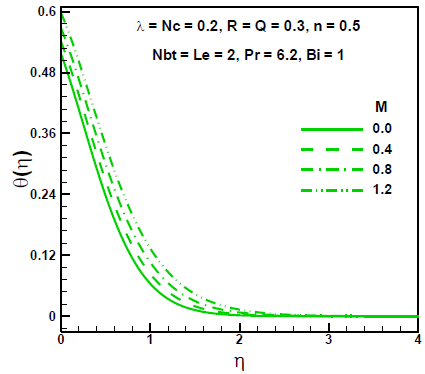Figure 2. Effects of M on dimensionless temperature

## 3.pngFigure 3. Effects of M on dimensionless concentration

## 4.png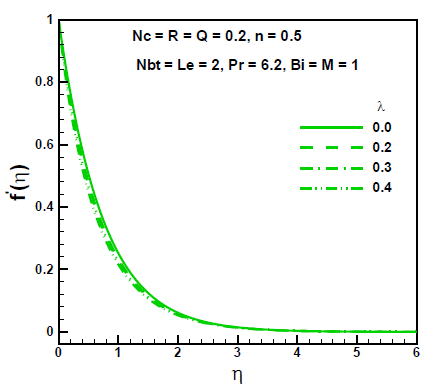Figure 4. Effects of λ on dimensionless velocity

## 5.pngFigure 5. Effects of λ on dimensionless temperature

## 6.png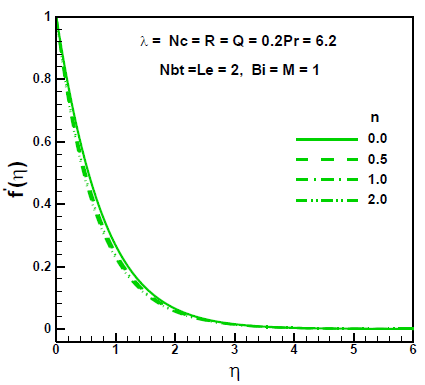Figure 6. Effects of n on dimensionless velocity

## 7.png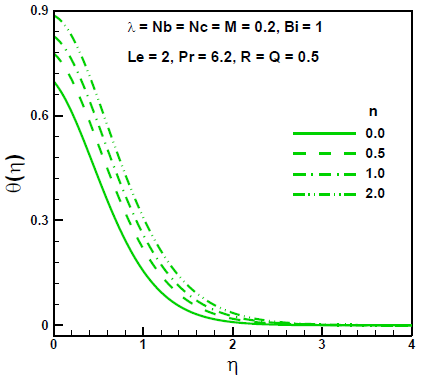Figure 7. Effects of n on dimensionless temperature

## 8.pngFigure 8. Effects of Bi on dimensionless temperature

## 9.pngFigure 9. Effects of Bi on dimensionless concentration

## 10.pngFigure 10. Effects of Nbt on dimensionless temperature

## 11.png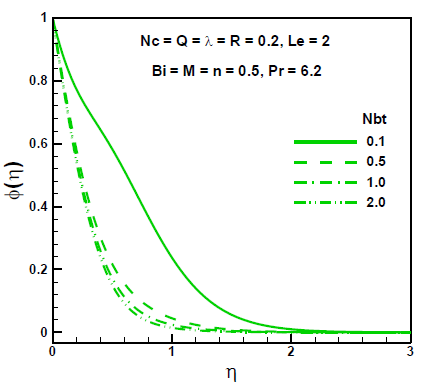Figure 11. Effects of Nbt on dimensionless concentration

## 12.png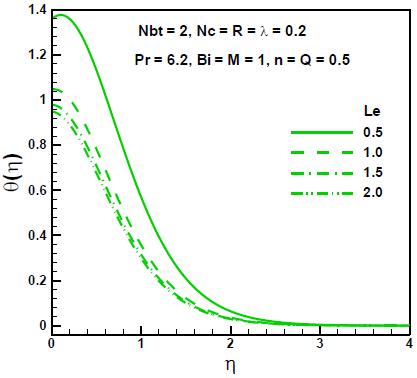Figure 12. Effects of Le on dimensionless temperature

## 13.png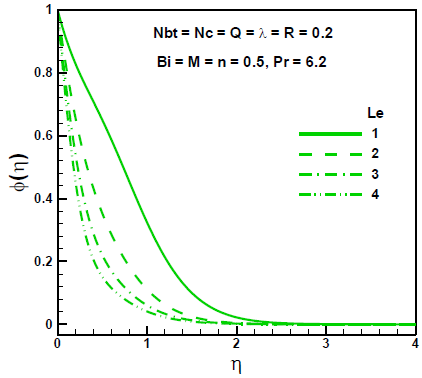Figure 13. Effects of Le on dimensionless concentration

## 14.pngFigure 14. Effects of Nc on dimensionless temperature

## 15.pngFigure 15. Effects of R on dimensionless temperature

## 16.png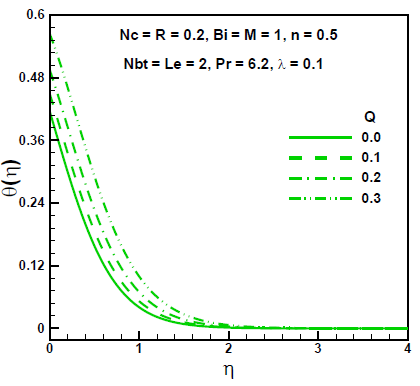Figure 16. Effects of Q on dimensionless temperature

## 17.pngFigure 17. Effects of M, λ and n on skin friction coefficient

## 18.png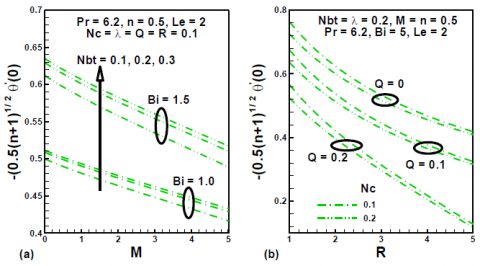Figure 18. Effects of M, Nb, Bi, Q, R and Nc on Nusselt number

## 19.pngFigure 19. Effects of M, λ and n on Sherwood number

6. Conclusion

The effects of thermal radiation and heat source on the MHD Williamson nanofluid flow over a non-linearly stretching surface with convective boundary conditions are investigated. The self-similar equations are obtained using similarity transformations. The self-similar equations are linearized by the quasilinearization technique and are then solved by finite difference method.  Mathematically it is appropriate to introduce product of Lewis number and Prandtl number instead of Schmidt number in the nanoparticles volumetric fraction equation. The study reveals that duo to increase of the magnetic field parameter and non-Newtonian Williamson parameter the momentum boundary layer thickness reduces and the thermal boundary layer thickness increases. The temperature as well as the thermal boundary layer thickness decrease with increasing values of diffusivity ratio. The effects of Lewis number and diffusivity ratio were found to decrease the temperature and concentration profiles. The skin friction factor increases with as the non-Newtonian Williamson parameter and magnetic field parameter increase. Nusselt number is increase with Biot number and decrease with the radiation and heat source parameters. Both heat source and heat capacities ratio tend to enhance Sherwood number.

Nomenclature

a      Stretching parameter

B      Magnetic field ( N/(mA))

b      Body force (N)

Bi     Biot number

C      Nanoparticles volumetric fraction

c       Specific heat (J/kg K)

DB    Brownian diffusion coefficient (m²/s)

DT     Thermophoretic diffusion coefficient (m²/s)

E        Electric field (N/C)

h        Convective heat transfer coefficient (W/m² K)

I        Identity tensor

J        Current density (A/m²)

i,j       Indexing variable

k        Nanofluid thermal conductivity (W/m K)

${{k}^{*}}$         mean absorption coefficient

M        Magnetic parameter

n         Stretching index

Nu      Local Nusselt number

p         Pressure (N/m²)

Pr        Prandtl number

${{Q}^{*}}$        heat generation constant

Q       heat generation parameter

Re      Local Reynolds number

S        Cauchy stress tensor (N/m²)

Sc       Schmidt number

Sh       Local Sherwood number

T         Temperature of fluid (K)

t         Time (s)

u        Horizontal component of velocity (m/s)

V        Velocity vector (m/s)

v        Vertical component of velocity (m/s)

x        Distance along the plate

Greek symbols

σ         Electrical conductivity (S/m)

${{\sigma }^{*}}$         Stefan–Boltzmann constant

ν         Kinematic viscosity (m²/s)

α         Thermal diffusivity (m²/s)

ρ         Density (kg/m³)

η         Similarity variable

λ         Non Newtonian Williamson parameter

Subscripts

B        Brownian motion,

T        Thermophoresis

∞        Infinity,

p         nanoparticles,

w        Wall

m        Iteration number

References

 Hayat T., Hussain Q., Javed T. (2009). The modified decomposition method and Pad´e approximants for the MHD flow over a non-linear stretching sheet, Nonlinear Analysis: Real World Applications, Vol. 10, pp. 966-973.

 Zheng L., Liu Y., Zhang X. (2012). Slip effects on MHD flow of a generalized Oldroyd-B fluid with fractional derivative, Non-Linear Anal: Real World Appl, Vol. 13,  pp. 513−523.

 Mabood F., Khan W.A., Ismail A.I.M. (2015). Approximate analytical modelling of heat and mass transfer in hydromagnetic flow over a non-isothermal stretched surface with heat generation/absorption and transpiration, Journal of the Taiwan Institute of Chemical Engineers, Vol. 54, pp. 11–19.

 Aman F., Ishak A. (2010). Hydromagnetic flow and heat transfer to a stretching sheet with prescribed surface heat flux, Heat Mass Transfer, Vol. 46, pp. 615–620.

 Ibrahim S.M., Reddy N.B. (2012). Radiation and mass transfer effects on MHD free convection flow along a stretching surface with viscous dissipation and heat generation, Int. J. of Appl. Math and Mech, Vol. 8, No. 8, pp. 1-21.

 Mabood F., Khan W.A., Ismail A.I.M. (2015). MHD stagnation point flow and heat transfer impinging on stretching sheet with chemical reaction and transpiration, Chemical Engineering Journal, Vol. 273, pp. 430–437.

 Mabood F., Mastroberardino A. (2015). Meting heat transfer on MHD convective flow of a nanofluid over a stretching sheet with viscous dissipation and second order slip, Journal of the Taiwan Institute of Chemical Engineers, pp. 1–7.

 Khan W.A., Pop I. (2010). Boundary layer flow of a nanofluid past a stretching sheet, Int. J. Heat Mass Transfer, Vol. 53, pp. 2477-2483.

 Rana P., Bhargava R. (2012). Flow and heat transfer of a nanofluid over a nonlinearly stretching sheet: a numerical study, Commun. Nonlinear Sci. Numer. Simul., Vol. 17, pp. 212-226.

 Mabood F., Khan W.A., Ismail A.I.M. (2015). MHD boundary layer flow and heat transfer of nanofluids over a nonlinear stretching sheet: a numerical study, Journal of Magnetism and Magnetic Materials, Vol. 374, pp. 569–576.

 Makinde O.D., Aziz A. (2011). Boundary layer flow of a nanofluid past a stretching sheet with a convective boundary convective conditions, Int. J. Therm.Sci., Vol. 50, pp. 1326–1332.

 Mabood F., Ibrahim S.M., Rashidi M.M., Shadloo M.S., Lorenzini G. (2016). Non-uniform heat source/sink and Soret effects on MHD non-Darcian convective flow past a stretching sheet in a micropolar fluid with radiation, Int. J. Heat Mass Transf, Vol. 93, pp. 674–682.

 Cortell R. (2007). Viscous flow and heat transfer over a nonlinearly stretching sheet, Appl. Math. Comput., Vol. 184,  pp. 864-873.

 Zaimi K., Ishak A., Pop I. (2014). Boundary layer flow and heat transfer over a nonlinearly permeable stretching/shrinking sheet in a nanofluid, Scientific Report 4, pp. 4404. DOI: 10. 1038/srep04404

 Sivakumar A., Alagumurthi N., Senthilvelan T. (2015). Experimental and numberical investigation of forced convective heat transfer coefficient in nanofluieds of Al2O3/water and CuO/EG in a serpentine shaped microchammel heat sink, International Journal of heat and Technology, Vol. 33, No. 1, pp. 155 – 160. DOI: 10.18280/ijht.330121

 Rajput G.R., Krishnaprasad J.S.V.R., Timol M.G. (2016). Group theoretic technique for MHD forced convection laminar boundary layer flow of nanofluid over a moving surface, International Journal of heat and Technology, Vol. 34, No. 1, pp. 1 –6, DOI: 10.18280/ijht.340101

 Fetecau C., Akhtar W., Imran M.A., Vieru D. (2010). On the oscillating motion of an Oldroyd-B fluid between two infinite circular cylinders, Comp. Math. Appl. Vol. 59, pp. 2836- 2845.

 Ellahi R., Aziz S., Zeeshan A. (2013). Non-Newtonian nanofluid flow through a porous medium between two co axial cylinders with heat transfer and variable viscosity, J. Porous Media, Vol. 16, No. 3, pp. 205-216.

 Abbasbandy S., Yurusoy M., Gulluce H. (2014). Analytical solutions of non-linear equations of power-law fluids of second grade over an infinite porous plate, Math. Comp. Appl., Vol. 19, No. 2, pp. 124.

 Rashidi M.M., Ali M., Freidoonimehr N., Rostami B., Hossian A. (2014). Mixed convection heat transfer for viscoelastic fluid flow over a porous wedge with thermal radiation, Adva.Mech. Eng., Vol. 204, pp. 735939.

 Hayat T., Imtiaz M., Alsaedi A. (2015). Effects of homogeneous-heterogeneous reactions in flow of Powell-Eyring fluid, J, Central South Uni, Vol. 22, pp. 3211−3216.

 Sheikholeslami M., Rashidi M.M. (2015). Ferrofluid heat transfer treatment in existence of variable magnetic field, The European Phys. J. Plus, Vol. 130, pp. 115.

 Hayat T., Asad S., Alsaedi A. (2015). Analysis for flow of Jeffrey fluid with nanoparticles, Chin. Phy. B, Vol. 23, No, 4, pp. 044702.

 Williamson R.V. (1929). The flow of pesudoplastic materials, Industrial & Engineering Chemistry Research, Vol. 21, pp. 1108.

 Irene D., Giambattista S. (2007). Perturbation solution for pulsatile flow of a non-Newtonian Williamson fluid in rock fracture, Mech. Min.Sci., Vol. 44, pp. 271–278.

 Nadeem S., Akram S. (2010). Influence of inclined magnetic field on peristaltic flow of a Williamson fluid model in an inclined symmetric or asymmetric channel, Math. Comp Model, Vol. 52, pp. 107-119.

 Vajravelu K., Sreenadh S., Rajanikanth K., Lee C. (2012). Peristaltic transport of a Williamson fluid in asymmetric channels with permeable walls, Nonlinear Analysis; Real World Applications, Vol. 13, pp. 2804–2822.

 Nadeem S., Akram S. (2010). Peristaltic flow of a Williamson fluid in an asymmetric channel, Comm. Nonlinear Sci. and Num. Simul., Vol. 15, pp. 1705–1716.

 Buongiorno J. (2006). Convective transport in nanofluids, J. Heat Transf., Vol. 128, pp. 240-250.

 Dapra S.G. (2007). Perturbation solution for pulsatile flow of a non Newtonian Williamson fluid in a rock fracture, Int J Rock Mech Min Sci., Vol. 44, pp. 271-278.

 Inoyue K., Tate A. (1974). Finite difference version of quasilinearization applied to boundary layer equations, AIAA J., pp. 558-560.

 Bellman R.E., Kalaba R.E. (1965). Quasilinearization and non-linear boundary value problems, America Elsevier Publishing Co. Inc., New Toyk.